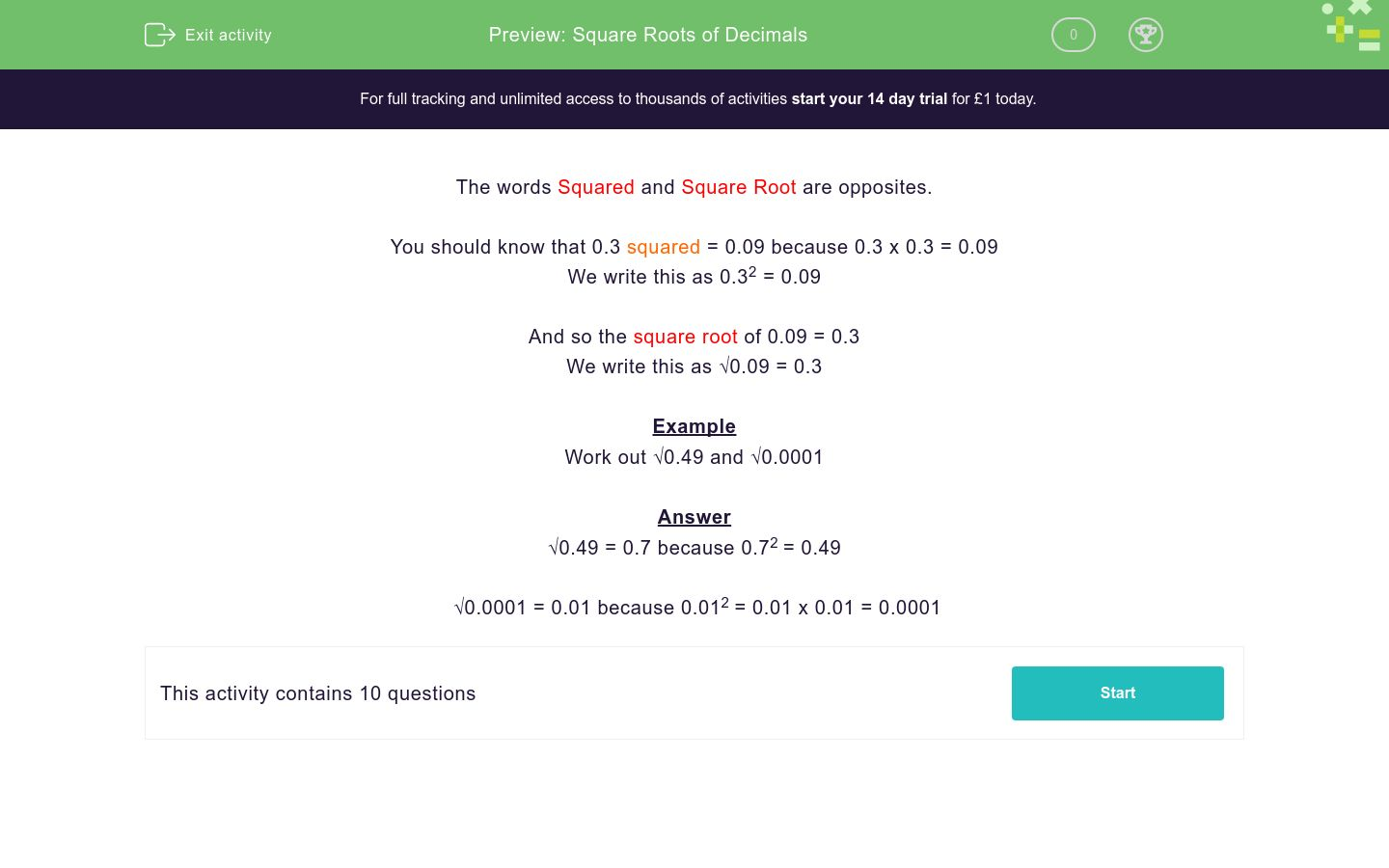# Square Roots of Decimals

In this worksheet, students state the square roots of decimal numbers.Key stage:  KS 3

Curriculum topic:   Number

Curriculum subtopic:   Understand Integer Powers/Real Roots

Difficulty level:### QUESTION 1 of 10

The words Squared and Square Root are opposites.

You should know that 0.3 squared = 0.09 because 0.3 x 0.3 = 0.09

We write this as 0.32 = 0.09

And so the square root of 0.09 = 0.3

We write this as √0.09 = 0.3

Example

Work out √0.49 and √0.0001

√0.49 = 0.7 because 0.72 = 0.49

√0.0001 = 0.01 because 0.01= 0.01 x 0.01 = 0.0001

Work out:

√0.01

Work out:

√0.16

Work out:

√0.09

Work out:

√0.49

Work out:

√0.81

Work out:

√0.25

Work out:

√0.36

Work out:

√0.64

Work out:

√0.0004

Work out:

√0.0009

• Question 1

Work out:

√0.01

0.1
EDDIE SAYS
What number must I multiply by itself to get 0.01?
• Question 2

Work out:

√0.16

0.4
EDDIE SAYS
What number must I multiply by itself to get 0.16?
• Question 3

Work out:

√0.09

0.3
EDDIE SAYS
What number must I multiply by itself to get 0.09?
• Question 4

Work out:

√0.49

0.7
EDDIE SAYS
What number must I multiply by itself to get 0.49?
• Question 5

Work out:

√0.81

0.9
EDDIE SAYS
What number must I multiply by itself to get 0.81?
• Question 6

Work out:

√0.25

0.5
EDDIE SAYS
What number must I multiply by itself to get 0.25?
• Question 7

Work out:

√0.36

0.6
EDDIE SAYS
What number must I multiply by itself to get 0.36?
• Question 8

Work out:

√0.64

0.8
EDDIE SAYS
What number must I multiply by itself to get 0.64?
• Question 9

Work out:

√0.0004

0.02
EDDIE SAYS
What number must I multiply by itself to get 0.0004?
• Question 10

Work out:

√0.0009

0.03
EDDIE SAYS
What number must I multiply by itself to get 0.0009?
---- OR ----